Question

# A separately excited DC motor has the following data P=32kW (rated power); Va=220V (rated voltage); Ia=171A...

A separately excited DC motor has the following data

P=32kW (rated power); Va=220V (rated voltage); Ia=171A (rated curent); n=1000 rpm (rated speed); Ra = 0.096 ohm

a.Calculate the armature voltage to reduce the speed to 900 rpm, assuming the load torque is kept constant?

b.Calculate the resistance should be added to armature circuit to reduce the speed to 800 rpm, assuming the load torque is kept constant?

c.Calculate the reduction of magnetic flux to reach the speed of 1100 rpm, assuming the load torque is kept constant?

d.Calculate the armature voltage to reduce the speed to 900 rpm, assuming the load torque is 90% rated torque?

e.Calculate the resistance should be added to armature circuit to reduce the speed to 800 rpm, assuming the load torque is 90% rated torque?

f.Calculate the reduction of magnetic flux to reach the speed of 1100 rpm, assuming the load torque is 90% rated torque?

We need at least 10 more requests to produce the answer.

0 / 10 have requested this problem solution

The more requests, the faster the answer.

All students who have requested the answer will be notified once they are available.

#### Earn Coins

Coins can be redeemed for fabulous gifts.

Similar Homework Help Questions
• ### A 230 V, 1000 rpm, 30 A separately excited motor has armature resistance and inductance of...

A 230 V, 1000 rpm, 30 A separately excited motor has armature resistance and inductance of 0.7 Ω and 50 mH respectively. Motor is controlled in regenerative braking by a chopper operating at 800 Hz from a dc source of 230 V. Assuming continuous conduction a) Calculate duty ratio of chopper for rated torque and the speed of 800 rpm. b) What will be the motor speed for duty ratio of 0.6 and rated motor torque? c) What will be...

• ### A shunt de motor is rated at 6kW, 120V and 1200 r/min has a magnetization curve...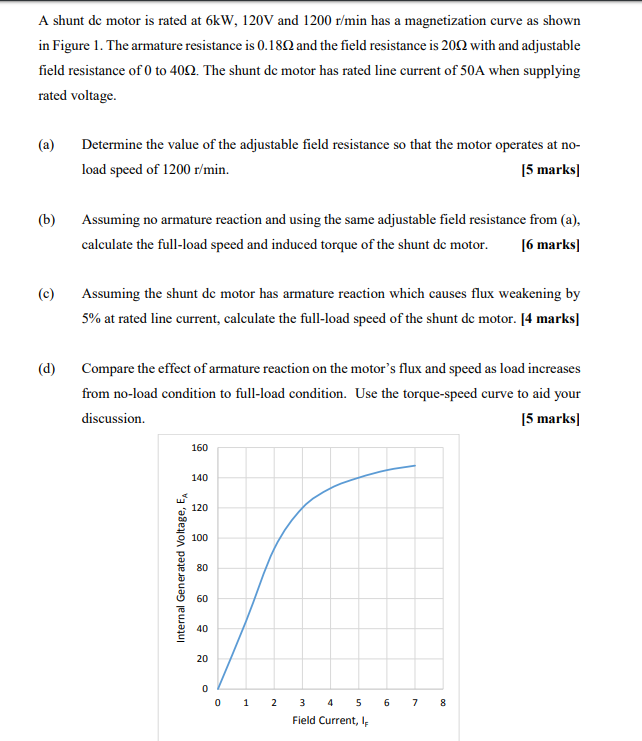A shunt de motor is rated at 6kW, 120V and 1200 r/min has a magnetization curve as shown in Figure 1. The armature resistance is 0.189 and the field resistance is 2002 with and adjustable field resistance of 0 to 4002. The shunt de motor has rated line current of 50A when supplying rated voltage. (a) Determine the value of the adjustable field resistance so that the motor operates at no- load speed of 1200 r/min. [5 marks] (b) Assuming...

• ### The permanent DC motor model CDP3450 by ABB is rated at 1 Hp, 2 500 RMP...

The permanent DC motor model CDP3450 by ABB is rated at 1 Hp, 2 500 RMP at 90v (no load). The armature resistance is 0.425 Ohm. a. The continuous current rating of this motor is 9.7 Amp, what is the maximum continuous torque rating? b. The motor is to drive a load of 2NM torque while maintaining a constant speed of 2000 RPM. What is the required supply current and voltage? c. With 90v armature voltage, what is the maximum...

• ### Problem 2 A separately excited DC motor is characterized by the followng intormation Armature res...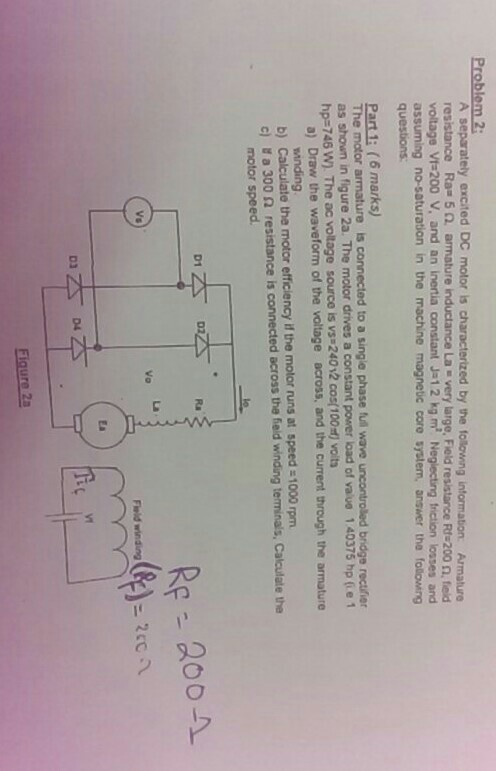Problem 2 A separately excited DC motor is characterized by the followng intormation Armature resistance Ra-5 2 armature inductance La . very large, Field resistance RS200 Ω, ted voltage Vt 200 V, and an inertia constant J-12 kg m Neglecting friction losses and assuming no-saturation in the machine magnetic core system, answer the folowing questions: Part 1: (6 marks) he motor a as shown in figure 2a. The motor drives a constant power load of vaue 1 40375 hp (ie...

• ### Given a 1/4 hp, separately excited, dc motor with the following parameters: K = 0.61, R, 15Ω, La-...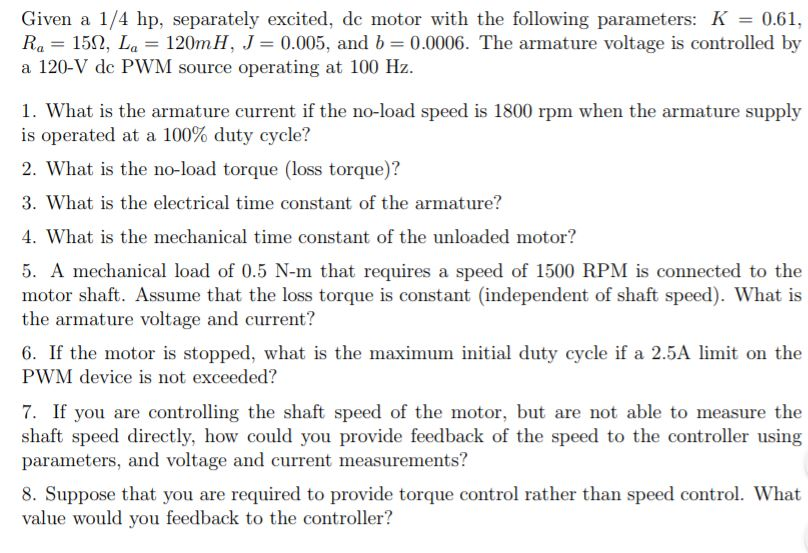I can not seem to get the right answers. Thank you! Given a 1/4 hp, separately excited, dc motor with the following parameters: K = 0.61, R, 15Ω, La-120mb. J = 0.005, and b = 0.0006. The armature voltage is controlled by a 120-V dc PWM source operating at 100 Hz 1. What is the armature current if the no-load speed is 1800 rpm when the armature supply is operated at a 100% duty cycle? 2. What is the no-load...

• ### (i) A 220 volts, 825 rpm, 125 A separately excited DC motor has an armature resistance...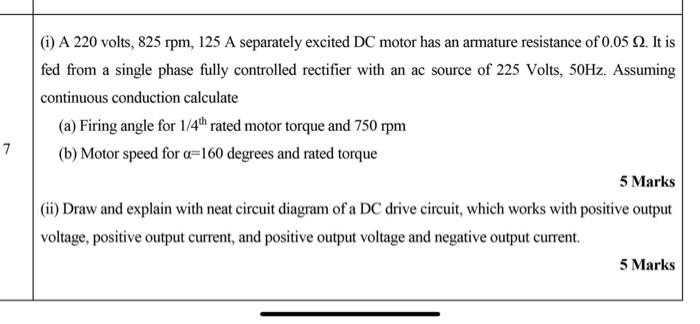(i) A 220 volts, 825 rpm, 125 A separately excited DC motor has an armature resistance of 0.05 2. It is fed from a single phase fully controlled rectifier with an ac source of 225 Volts, 50Hz. Assuming continuous conduction calculate (a) Firing angle for 1/4"hrated motor torque and 750 rpm (b) Motor speed for a=160 degrees and rated torque 5 Marks (ii) Draw and explain with neat circuit diagram of a DC drive circuit, which works with positive output...

• ### An eight-pole, 25 kW, 120 V DC generator has a duplex lap-wound armature, which has 64 coils with 16 turns per coil. Its rated speed is 2400 rpmQuesition a1. An eight-pole, 25 kW, 120 V DC generator has a duplex lap-wound armature, which has 64 coils with 16 turns per coil. Its rated speed is 2400 rpm.                                            (a)   How much flux per pole is required to produce the rated voltage in this generator at no load conditions?                                                                                        (b)   What is the induced torque in this machine at...

• ### An eight-pole, 25 kW, 120 V DC generator has a duplex lap-wound armature, which has 64 coils with 16 turns per coil. Its rated speed is 2400 rpm (HELP)Question:  An eight-pole, 25 kW, 120 V DC generator has a duplex lap-wound armature, which has 64 coils with 16 turns per coil. Its rated speed is 2400 rpm.(a)   Define a duplex lap-wound DC armature.                                                      (b)   How much flux per pole is required to produce the rated voltage in this generator at no load conditions?                                                                                        (c)   What is the induced torque in this machine at rated load?                            (d)   Determine path current in the armature of this generator at the rated load? ...

• ### A separately excited DC shunt generator produces a no-load voltage of 150 Vdc at 1200 RPM....

A separately excited DC shunt generator produces a no-load voltage of 150 Vdc at 1200 RPM. If the armature resistance is 3 Ohms, calculate the output voltage under the following conditions: (Note all calculations for each problem are to be performed from the base values given above). a) The speed is reduced to 875 RPM. Vout = __________________ b) The field current is increased by 22% (assume a linear field current/flux relationship). Vout = __________________ c) The speed is increased...

• ### A separately excited dc motor is fed by a 3-phase, 6-pulse converter shown in figure Q5. The 3-phase line is a 500 V, 6...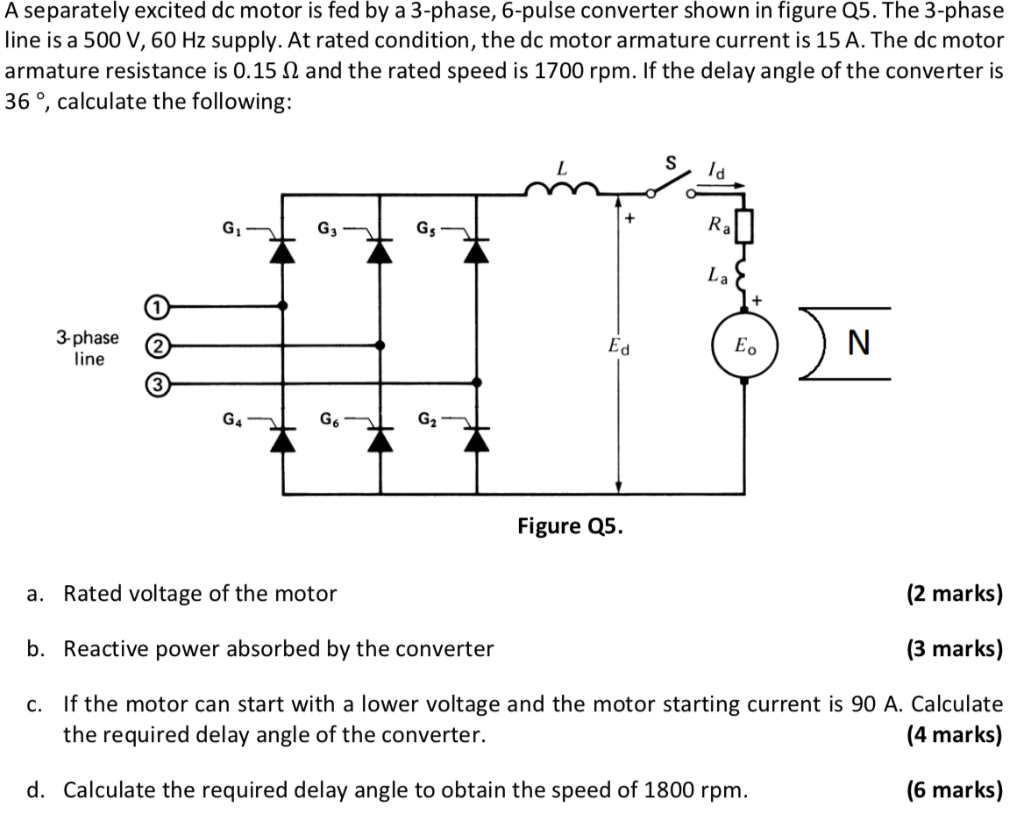A separately excited dc motor is fed by a 3-phase, 6-pulse converter shown in figure Q5. The 3-phase line is a 500 V, 60 Hz supply. At rated condition, the dc motor armature current is 15 A. The dc motor armature resistance is 0.15 Ω and the rated speed is 1700 rpm. If the delay angle of the converter is 36 °, calculate the following: 3-phase (2 0 line Figure Q5. (2 marks) Rated voltage of the motor a. b....